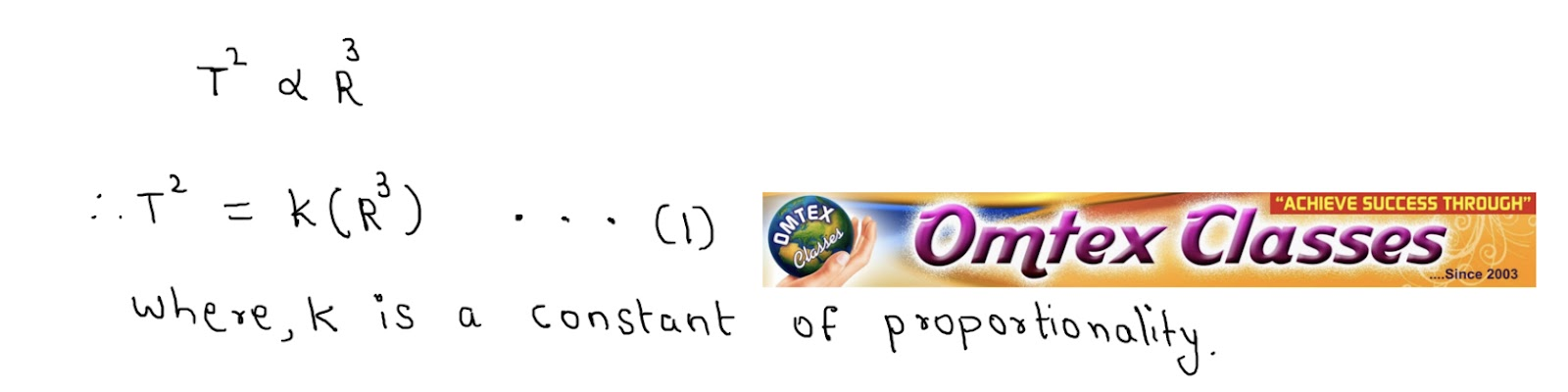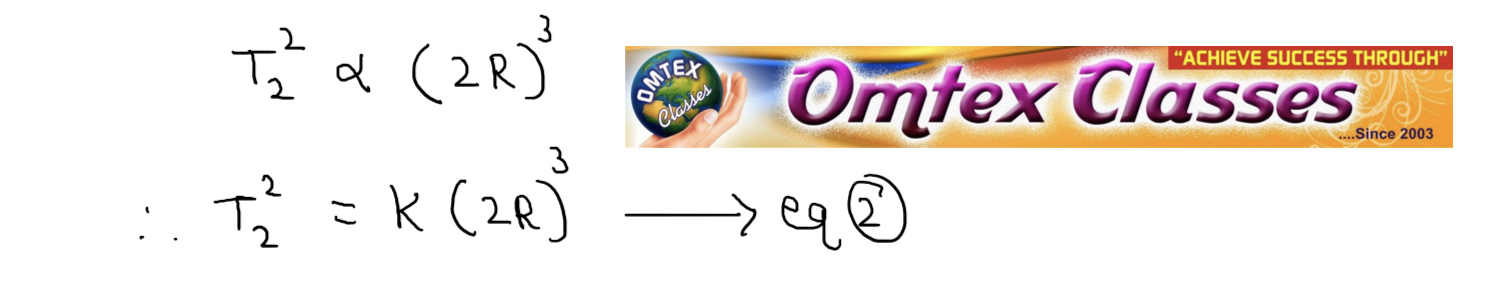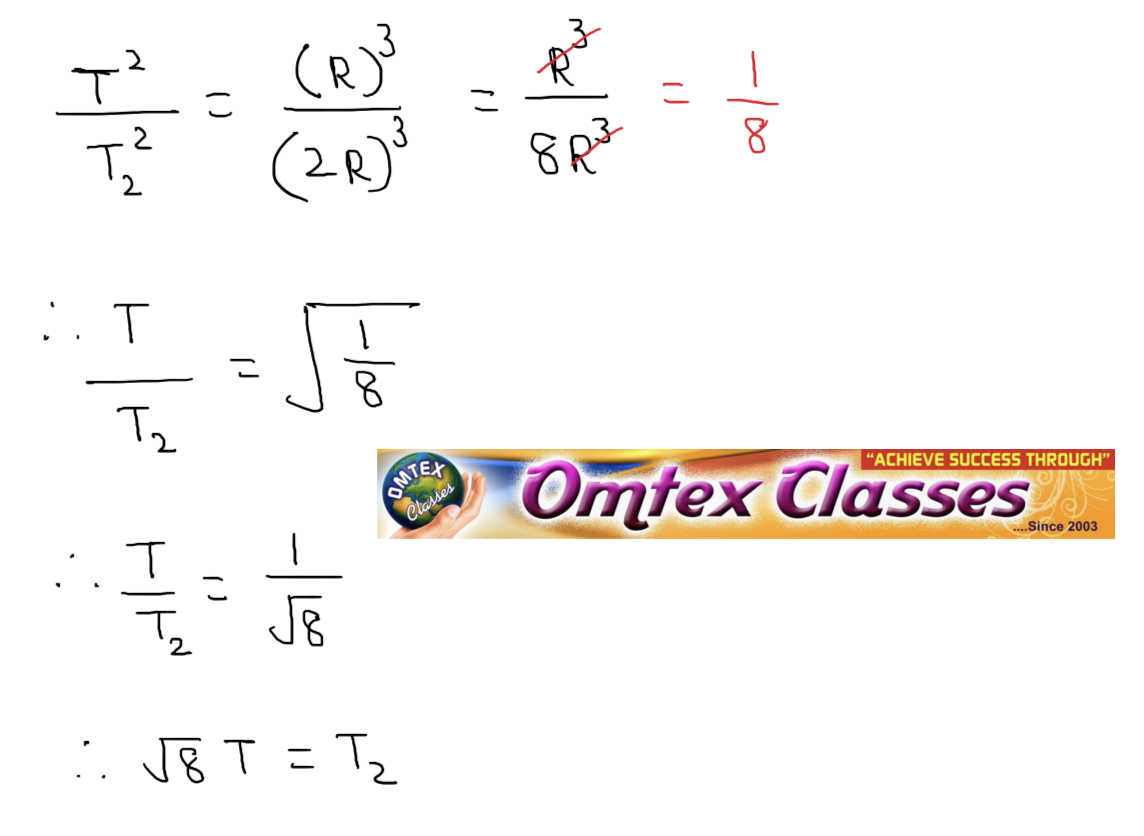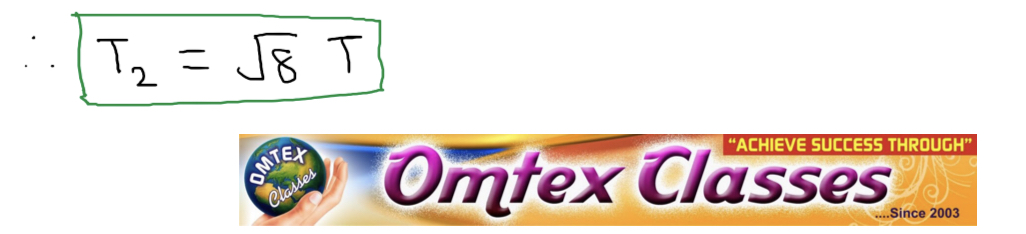SSC BOARD PAPERS IMPORTANT TOPICS COVERED FOR BOARD EXAM 2024

### Let the period of revolution of a planet at a distance R from a star be T. Prove that if it was at a distance of 2R from the star, its period of revolution will be √8 T.

4. Let the period of revolution of a planet at a distance R from a star be T. Prove that if it was at a distance of 2R from the star, its period of revolution will be √8 T.
Ans. i. According to Kepler’s third law, The square of orbital period of revolution T of a planet around a star is directly proportional to the cube of the mean distance R of the planet from the star.ii. When the planet, is at a distance of 2R from the star, then its period of revolution T2  will be,Iii. Dividing equation (1) and (2), we getThus, for a planet, at a distance of 2R from the star, its period of revolution will be √8 T.